• 按位运算符 c语言提供了6个位操作运算符。这些运算符只能作用于整数操作数，及只能作用于带符号或无符号的char，short，int，long类型。 按位与运算符& 运算机制：将操作数化为二进制后，比较各个位置上的0和1...
部分参考scdxmoe的博客
按位运算符
c语言提供了6个位操作运算符。这些运算符只能作用于整数操作数，及只能作用于带符号或无符号的char，short，int，long类型。

按位与运算符&
运算机制：将操作数化为二进制后，比较各个位置上的0和1,都为1则结果为1，其他情况为0.
例如：0 0 0 1 1 0 1 1
---------1 0 1 0 1 0 1 0
---------------------------------
结果： 0 0 0 0 1 0 1 0
负数用补码的方式计算。（计算后的结果记得取反得到真正的结果。）
应用：&常用于屏蔽某些二进制位，清零某个数或取得某位数的后X位。

按位或（|），异或运算符（^）
异或运算符：  两个操作数的对应位不相同时将该位置为1，否则为0。
或运算符：  两个操作数的对应位相同时将该位置为1，否则为0。
例如：0 0 0 1 1 0 1 1
---------1 0 1 0 1 0 1 0
---------------------------------
或------0 1 0 0 1 1 1 0
例如：0 0 0 1 1 0 1 1
---------1 0 1 0 1 0 1 0
---------------------------------
异或—1 0 1 1 0 0 0 1
按位或运算符 | 常用于将某些二进制位置为1.

“或运算”应用：
常用来对一个数据的某些位置1。
方法：找到一个数，对应X要置1的位，该数的对应位为1，其余位为零。此数与X相或可使X中的某些位置为1。
异或运算”应用：
使特定位翻转 找一个数，对应X要翻转的各位，该数的对应位为1，其余位为零，此数与X对应位异或即可。
  #include<stdio.h>

int main(){
int a=55;
int b=168;
printf("a&b=%d a|b=%d a^b=%d\n",a&b,a|b,a^b);

int c=-2;
char d='0';
printf("c&d=%d c|d=%d c^d=%d\n",c&d, c|d, c^d);
}



左移（<<），右移(>>)
将二进制的位数左移右移。
左移n位等价于乘2^n，同理，右移n位等于除2的n次方。
（a<<2=a*4,a>>2=a/4）

在对unsigned右移的时候，左边空出的部分用0填补。
在对signed右移的时候，某些机器将对左边空出的部分用符号填补（算术移位），而另一些机器则对左边空出的部分用0填补。（逻辑移位）
右移时，左补0 or 补1 得看被移数是正还是负。
#include<stdio.h>

int main(){
int a=55;
int b=-16;
printf("a<<1=%d a>>1=%d b<<1=%d b>>1=%d\n",a<<1, a>>1, b<<1, b>>1);

}


按位求反（～）
对一个二进制数按位取反，即将0变1，1变0。
按位求反是一元运算符，所以优先级最高。


展开全文• python 按位运算符Python bitwise operators are used to perform bitwise calculations on integers. The integers are converted into binary format and then operations are performed bit by bit, hence the ...
python 按位运算符Python bitwise operators are used to perform bitwise calculations on integers. The integers are converted into binary format and then operations are performed bit by bit, hence the name bitwise operators. Python按位运算符用于对整数执行按位计算。 整数转换为二进制格式，然后逐位执行运算，因此命名为按位运算符。
Python bitwise operators work on integers only and the final output is returned in the decimal format. Python bitwise operators are also called binary operators. Python按位运算符仅对整数起作用，并且最终输出以十进制格式返回。 Python按位运算符也称为二进制运算符。

Python按位运算符 (Python Bitwise Operators)
There are 6 bitwise operators in Python. The below table provides short details about them. Python中有6个按位运算符。 下表提供了有关它们的简短详细信息。
Bitwise OperatorDescriptionSimple Example&Bitwise AND Operator10 & 7 = 2|Bitwise OR Operator10 | 7 = 15^Bitwise XOR Operator10 ^ 7 = 13~Bitwise Ones’ Compliment Operator~10 = -11<<Bitwise Left Shift operator10<<2 = 40>>Bitwise Right Shift Operator10>>1 = 5

按位运算符
描述
简单的例子

和
按位与运算符
10＆7 = 2

|
按位或运算符
10 | 7 = 15

^
按位XOR运算符
10 ^ 7 = 13

〜
按位补码运算符
〜10 = -11

<<
按位左移运算符
10 << 2 = 40

>>
按位右移运算符
10 >> 1 = 5

Let’s look into these operators one by one and understand how they work. 让我们逐一研究这些运算符，并了解它们的工作方式。
1.按位与运算符 (1. Bitwise AND Operator)
Python bitwise and operator returns 1 if both the bits are 1, otherwise 0. 如果两个位均为1，Python按位运算符将返回1，否则返回0。
>>> 10&7
2
>>>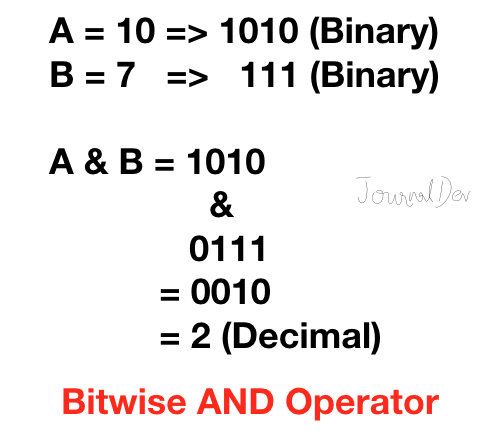Python Bitwise And Operator Python按位与运算符
2.按位或运算符 (2. Bitwise OR Operator)
Python bitwise or operator returns 1 if any of the bits is 1. If both the bits are 0, then it returns 0. 如果任何位为1，Python按位或运算符将返回1。如果两个位均为0，则它​​将返回0。
>>> 10|7
15
>>>Python Bitwise Or Operator Python按位或运算符
3.按位XOR运算符 (3. Bitwise XOR Operator)
Python bitwise XOR operator returns 1 if one of the bits is 0 and the other bit is 1. If both the bits are 0 or 1, then it returns 0. 如果位之一为0，另一位为1，则Python按位XOR运算符将返回1。如果两个位均为0或1，则它将返回0。
>>> 10^7
13
>>>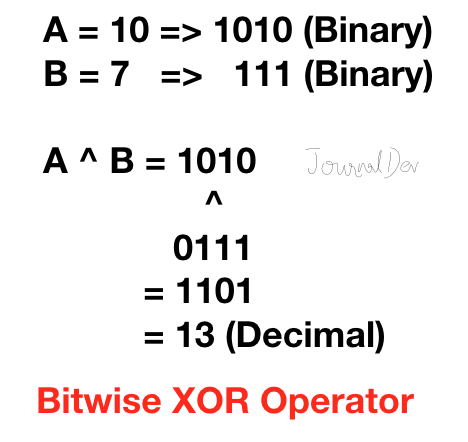Python Bitwise XOR Operator Python按位XOR运算符
4.按位补码运算符 (4. Bitwise Ones’ Complement Operator)
Python Ones’ complement of a number ‘A’ is equal to -(A+1). Python Ones的数字'A'的补码等于-（A + 1）。
>>> ~10
-11
>>> ~-10
9
>>>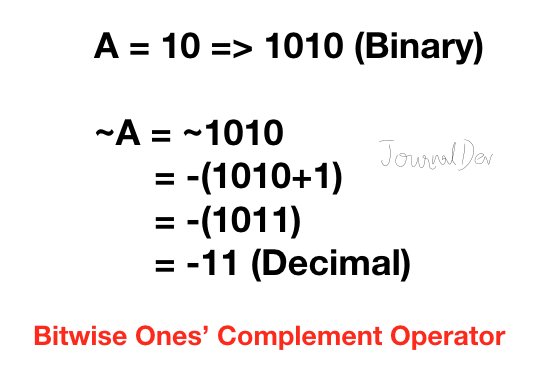Python Bitwise Ones Complement Operator Python逐位补码运算符
5.按位左移运算符 (5. Bitwise Left Shift Operator)
Python bitwise left shift operator shifts the left operand bits towards the left side for the given number of times in the right operand. In simple terms, the binary number is appended with 0s at the end. Python按位左移运算符将右操作数中的给定次数向左移动左操作数位。 简而言之，二进制数末尾带有0。
>>> 10 << 2
40
>>>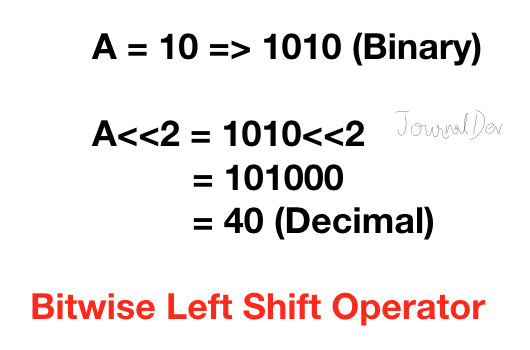Python Bitwise Left Shift Operator Python按位左移运算符
6.按位右移运算符 (6. Bitwise Right Shift Operator)
Python right shift operator is exactly the opposite of the left shift operator. Then left side operand bits are moved towards the right side for the given number of times. In simple terms, the right side bits are removed. Python的右移运算符与左移运算符完全相反。 然后将左侧操作数位向右侧移动给定次数。 简单来说，右侧位已删除。
>>> 10 >> 2
2
>>>Python Bitwise Right Shift Operator Python按位右移运算符
Python supports operator overloading. There are various methods that we can implement to support bitwise operators for our custom objects. Python支持运算符重载。 我们可以实现多种方法来支持自定义对象的按位运算符。
Bitwise OperatorMethod to Implement&__and__(self, other)|__or__(self, other)^__xor__(self, other)~__invert__(self)<<__lshift__(self, other)>>__rshift__(self, other)

按位运算符
实施方法

和
__和__（自己，其他）

|
__或__（自己，其他）

^
__xor __（自己，其他）

〜
__invert __（个体经营）

<<
__lshift __（自己，其他）

>>
__rshift __（自己，其他）

Here is an example of a bitwise operator overloading for our custom object. 这是我们的自定义对象按位运算符重载的示例。
class Data:
id = 0

def __init__(self, i):
self.id = i

def __and__(self, other):
if isinstance(other, Data):
return Data(self.id & other.id)
else:
raise ValueError('Argument must be object of Data')

def __or__(self, other):
if isinstance(other, Data):
return Data(self.id | other.id)
else:
raise ValueError('Argument must be object of Data')

def __xor__(self, other):
if isinstance(other, Data):
return Data(self.id ^ other.id)
else:
raise ValueError('Argument must be object of Data')

def __lshift__(self, other):
if isinstance(other, int):
return Data(self.id << other)
else:
raise ValueError('Argument must be integer')

def __rshift__(self, other):
if isinstance(other, int):
return Data(self.id >> other)
else:
raise ValueError('Argument must be integer')

def __invert__(self):
return Data(~self.id)

def __str__(self):
return f'Data[{self.id}]'

d1 = Data(10)
d2 = Data(7)

print(f'd1&d2 = {d1&d2}')
print(f'd1|d2 = {d1|d2}')
print(f'd1^d2 = {d1^d2}')
print(f'd1<<2 = {d1<<2}')
print(f'd1>>2 = {d1>>2}')
print(f'~d1 = {~d1}')Output: 输出：
Bitwise AND operator overloaded
d1&d2 = Data
d1|d2 = Data
d1^d2 = Data
d1<<2 = Data
d1>>2 = Data
~d1 = Data[-11]f-strings in Python.Python中的f-strings 。  摘要 (Summary)
Python bitwise operators are mostly used in mathematical calculations. We can implement specific methods to support bitwise operators for our custom class implementations too. Python按位运算符通常用于数学计算。 我们也可以实现特定的方法来为我们的自定义类实现支持按位运算符。
翻译自: https://www.journaldev.com/26737/python-bitwise-operatorspython 按位运算符
展开全文• Java教程 - Java按位运算符按位操作符作用于其操作数的各个位。Java按位运算符可以应用于整数types:long，int，short，char，byte。按位运算符列表下表列出了所有Java按位运算符。运算符 结果~ 按位一元NOT& 按...
Java教程 -  Java按位运算符按位操作符作用于其操作数的各个位。Java按位运算符可以应用于整数types:long，int，short，char，byte。按位运算符列表下表列出了所有Java按位运算符。运算符            结果~                   按位一元NOT&   按位AND|        按位或^        按位异或>>  向右移动>>> 右移零填充<<  向左移动&=  按位AND分配|=                  按位OR分配^=                  按位异OR分配>>= 右移赋值>>>=右移零填充分配<<= 向左移位按位运算符分配将赋值与按位运算组合。以下两个语句是等效的:a = a >> 4;a >>= 4;以下两个语句是等效的:a = a | b;a |= b;以下程序演示了按位运算符分配:public class Main {public static void main(String args[]) {int a = 1;int b = 2;int c = 3;a |= 2;b >>= 2;c <<= 2;a ^= c;System.out.println("a = " + a);System.out.println("b = " + b);System.out.println("c = " + c);}}此程序的输出如下所示: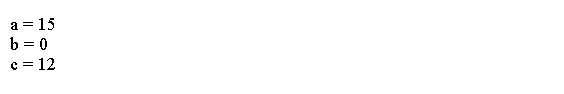Java左移运算符左移位算子，<它有这种一般形式:value << num以下代码移位字节类型变量。public class Main {public static void main(String args[]) {byte a = 64, b;int i;i = a << 2;b = (byte) (a << 2);System.out.println("Original value of a: " + a);System.out.println("i and b: " + i + " " + b);}}此程序生成的输出如下所示:例子每个左移具有使原始值加倍的效果。以下程序说明了这一点:public class Main {public static void main(String args[]) {int num = 0xFFFFFFF;for (int i = 0; i < 4; i++) {num = num << 1;System.out.println(num);}}}程序生成以下输出: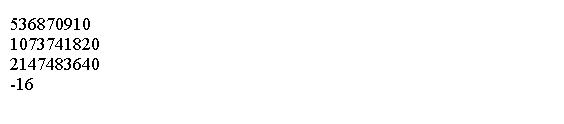Java右移运算符右移位运算符>>将值中的所有位向右移a指定次数。其一般形式如下所示:value >> numnum指定要向右移位的位置数。以下代码段将值32向右移两个位置:public class Main {public static void main(String[] argv) {int a = 32;a = a >> 2;System.out.println("a is " + a);}}输出: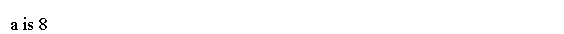Java无符号右移Java无符号，右移运算符，>>>，始终将零转移到高阶位。其一般形式如下所示:value >>> numnum指定要向右移位的位置数。以下代码显示如何使用无符号右移。public class Main {public static void main(String[] argv) {int a = -1;a = a >>> 24;System.out.println("a is " + a);}}输出: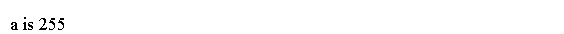展开全文• 按位运算符是把数字看作二进制来进行计算的。Python中的按位运算法则如下：按位与 ( bitwise and of x and y )& 举例： 5&3 = 1 解释： 101 11 相同位仅为个位1 ，故结果为 1按位或 ( bitwise or of x and y...
按位运算符是把数字看作二进制来进行计算的。Python中的按位运算法则如下：按位与 ( bitwise and of x and y )& 举例： 5&3 = 1 解释： 101 11 相同位仅为个位1 ，故结果为 1按位或 ( bitwise or of x and y )| 举例： 5|3 = 7 解释： 101 11 出现1的位是 1 1 1，故结果为 111按位异或 ( bitwise exclusive or of x and y )^ 举例： 5^3 = 6 解释： 101 11 对位相加(不进位)是 1 1 0，故结果为 110按位反转 (the bits of x inverted )~ 举例： ~5 = -6 解释： 将二进制数+1之后乘以-1，即~x = -(x+1)，-(101 + 1) = -110按位反转仅能用在数字前面。所以写成 3+~5 可以得到结果-3，写成3~5就出错了按位左移 ( x shifted left by n bits )<< 举例: 5<<2 = 20 解释：101 向左移动2位得到 10100 ，即右面多出2位用0补按位右移 ( x shifted right by n bits )>> 举例： 5>>2 = 1 解释：101 向右移动2位得到 1，即去掉右面的2位八进制，Octal，缩写OCT或O，一种以8为基数的计数法，采用0，1，2，3，4，5，6，7八个数字，逢八进1。一些编程语言中常常以数字0开始表明该数字是八进制。八进制的数和二进制数可以按位对应(八进制一位对应二进制三位)，因此常应用在计算机语言中。十六进制(英文名称：Hexadecimal)，是计算机中数据的一种表示方法。同我们日常生活中的表示法不一样。它由0-9，A-F组成，字母不区分大小写。与10进制的对应关系是：0-9对应0-9;A-F对应10-15;N进制的数可以用0~(N-1)的数表示，超过9的用字母A-F。正数的源码与补码、反码一致;负数的反码是将其源码除符号位之外的各位求反，负数的补码是将其源码出符号位之外的各位求反后再加1。如您对本文有疑问或者有任何想说的，请点击进行留言回复，万千网友为您解惑！
展开全文• 按位运算符是把数字看作二进制来进行计算的。Python中的按位运算法则如下：按位与 ( bitwise and of x and y )& 举例： 5&3 = 1 解释： 101 11 相同位仅为个位1 ，故结果为 1按位或 ( bitwise or of x and y...
• 按位运算符是把数字看作二进制来进行计算的。Python中的按位运算法则如下：按位与 ( bitwise and of x and y )举例： 53 = 1 解释： 101 11 相同位仅为个位1 ，故结果为 1按位或 ( bitwise or of x and y )| 举例： ...
• Verilog逻辑运算符与按位运算符： 区别：按位运算符进行逐位的逻辑运算（如：与或非），输出与输入位数一致； 逻辑运算符进行逻辑运算，不关注输入的某一位而是将输入作为整体进行逻辑操作，输出位数为1； 列举：...fpga verilog
• 关系运算符①关系运算符等级②逻辑运算符③按位运算符总结 ①关系运算符等级 a. > < b. >= <= c. == != 可以用来比较大小 长短等 ②逻辑运算符 ③按位运算符 总结 ...
• 按位运算符是把数字看作二进制来进行计算的。Python中的按位运算法则如下：按位与 ( bitwise and of x and y )& 举例： 5&3 = 1 解释： 101 11 相同位仅为个位1 ，故结果为 1按位或 ( bitwise or of x and y...
• 按位运算符是把数字看作二进制来进行计算的。Python中的按位运算法则如下：按位与 ( bitwise and of x and y )& 举例： 5&3 = 1 解释： 101 11 相同位仅为个位1 ，故结果为 1按位或 ( bitwise or of x and y...
• 布尔运算符与按位运算符我对何时应该使用布尔运算符与按位运算符感到困惑and vs &, or vs |有人能启发我何时使用每种药物，何时使用一种药物会影响我的结果？8个解决方案56 votes以下是一些准则：布尔运算符通常...
• 大多数语言都提供了按位运算符按位运算符在c,c++等语言中运用广泛，而在JS，AS等脚本语言中则没有太多的应用例子，有时候，适当的使用按位运算符会取得很好的效果。下面根据自己的认知简单的谈一下js中的位操作...
• 按位运算符 按位运算符有六个： & 按位与（AND） | 按位或（OR） ^ 按位异或（EOR） ~ 取反（NOT） >> 右移...
• C 按位运算符分为以下两种： <1> 按位逻辑运算符 <2> 移位运算符 其中 ，按位逻辑运算符分为以下4种： (1) 二进制反码或按位取反: ~ 分析： 一元运算符 ~ 把1变为0， 把0变为1。例如： ~（10011010）//...程序人生
• 1、Python 按位运算符按位运算符用于比较(二进制)数字：运算符名称描述&按位与运算符(AND)如果两个位均为1，则将每个位设置为1|按位或运算符(OR)如果两位之一为1，则将每个位设置为1^按位异或运算符(XOR)如果两...
• java按位运算符（操作符）         最近在看源码时，发现一些java基础有些记不清了。今天来回忆，整理一下。         按位运算符是来操作整数基本数据类型中的...
• 下面主要以按位运算符为例。 按位逻辑运算符 4个按位逻辑运算符都用于整形数据，包括char。之所以叫作按位运算符，是因为这些操作都是针对每一个位进行，不影响在左右两边的位。不要把这些运算符与常规运算符(&......